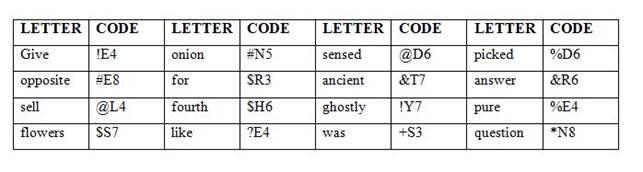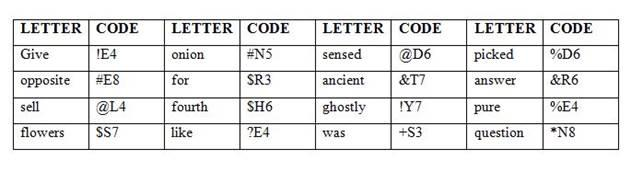## Coding and Decoding For SBI PO : Set – 09

D.1-5) Study the following information carefully and answer the questions which follow—In a certain code language, some statements are coded as follow:

Give money some can” coded as ‘&a3 @i4 *o5 %o4’

”Camel and time gold” is coded as ‘&a5 #i4 \$n3 @o4’

Access sound target see” is coded as ‘%e3 %o5 #a6 \$c6’

Match around go more” is coded as ‘\$r6 *a5 @o2 *o4’.

1) What will be the code for ‘Mobile sound’?

a) *o5 %o6

b) %m4 @o6

c) *o5 %s6

d) %o6 #m7

e) None of these

e) Mobile sound” is coded as        –        *o6    %o5

From the above arrangements ‘Give’ is coded as ‘@i4’ this is coded by ‘G’ is coded as ‘@’and ‘i’ is second letter of the word. ‘4’ is the Total number of letters present in the given word. In this same way all the coding are arranged.

G @

C &

T #

S %

M *

A \$

2) What will be the code for ‘money time go’?

a) *o5 #i4 @g2

b) #i4 @o2 *m5

c) #i4 @o2 *o5

d) \$i5 @o2 %t4

e) None of these

c)

Money time go” is coded as      –        #i4    @o2   *o5

From the above arrangements ‘Give’ is coded as ‘@i4’ this is coded by ‘G’ is coded as ‘@’and ‘i’ is second letter of the word. ‘4’ is the Total number of letters present in the given word. In this same way all the coding are arranged.

G @

C &

T #

S %

M *

A \$

3) Which of the following will be the code for ‘Session card’?

a) #a4 *e7

b) &c4 %s7

c) &a4 %e7

d) &a7 %a4

e) None of these

c)

Session card” is coded as                  –        &a4    %e7

From the above arrangements ‘Give’ is coded as ‘@i4’ this is coded by ‘G’ is coded as ‘@’and ‘i’ is second letter of the word. ‘4’ is the Total number of letters present in the given word. In this same way all the coding are arranged.

G @

C &

T #

S %

M *

A \$

4) What will be the code for ‘Match’?

a) *a5

b) @o2

c) %m5

d) *o5

e) None of these

a)

Match” is coded as                    –        *a5

From the above arrangements ‘Give’ is coded as ‘@i4’ this is coded by ‘G’ is coded as ‘@’and ‘i’ is second letter of the word. ‘4’ is the Total number of letters present in the given word. In this same way all the coding are arranged.

G @

C &

T #

S %

M *

A \$

5) Which of the following will be the code for ‘More camel’?

a) *a5 *o4

b) *a4 &o5

c) @o5 #a6

d) *o4 &a5

e) @a5 #o3

d)

More camel” is coded as           –        *o4    &a5

D.6-10) study the following information carefully and answer the following questions which follows certain code language.

Give answer for question” is coded as \$R3 &R6 *N8 !E4

opposite pure fourth sensed” is coded as @D6 \$A6 #E8 %E4

Sell flowers like ancient ” is coded as ?E4 &T7 @L4 \$S7

picked onion was ghostly” #N5 !Y7 %D6 +S3

6) If “Give like most“ is coded as ?E4 ^T4 !E4, then “Most answer south” is coded as?

a) &R6 \$H6 ^T4

b) @D6 \$D6 \$H5

c) ^T4 &R6 @H5

d) ^T4 @D6 #N5

e) None of these.

c)

Symbol=A symbol represents individually for starting letter of the word.

Letter= Last letter of each word

Number= Count of letter in each word.most=^T4  answer=&R6   South = @H5

7) what will be the code for ‘picked sensed’

a) \$H6 @D6

b) !Y7 %D6

c) %D6 @D6

d) %D6 #E8

e) @D6 \$D6

c)

Symbol=A symbol represents individually for starting letter of the word.

Letter= Last letter of each word

Number= Count of letter in each word.Picked=%D6, Sensed=@

8) what will be the code for pure ?

a) @D6

b) %P4

c) @L4

d) %E4

e) ?E4

d)

Symbol=A symbol represents individually for starting letter of the word.

Letter= Last letter of each word

Number= Count of letter in each word.Pure=%E4

9) what will be the code for “pure onion healthy”?

a) #N5 !E4 ^Y7

b) ~Y7 %E4 #N5

c) %E4 !Y7 #N5

d) %E4 &R6 %D6

e) #N5 ~Y6 %E4

b)

Symbol=A symbol represents individually for starting letter of the word.

Letter= Last letter of each word

Number= Count of letter in each word.Pure =%E4, Onion=#N5, Healthy=~Y7

10) what will be the code for flowers?

a) &T7

b) \$S6

c) !Y7

d) \$S7

e) @L4Flowers is coded as \$S7